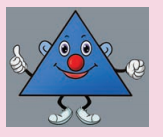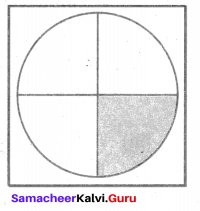## Tamilnadu Samacheer Kalvi 6th Maths Solutions Term 3 Chapter 5 Information Processing Intext Questions

Exercise 5.1

Try These (Text book Page No. 74)

Question 1.
Find the 10th term of the Fibonacci sequence
Solution:
We have the Fibonacci sequence 1, 1, 2, 3, 5, 8, 13, 21, 34, 55,….
The 10th term is 55.

Question 2.
If the 11th term of the Fibonacci sequence is 89 and 13th term is 233 then, what is the 12th term?
Solution:
Given 11th term of the Fibonacci sequence is 89 and 13th term of is 233
We know that a number in Fibonacci sequence is got by adding the previous two consecutive numbers.
11th term + 12th term = 13th term
89 +12th term =233
∴ 12th term = 233 – 89 = 144
∴ 12th term in Fibonacci sequence = 144.

Try this (Text book Page No.75)

Question 1.
Are two consecutive Fibonacci numbers relatively prime?
Solution:
GCD of two consecutive numbers in Fibonacci sequence is 1.
∴ They are relatively prime.Try These (Text book Page No. 78)

Question 1.
The teacher should give oral instructions to the students to draw the geometrical figure already drawn by him / her.
i) Draw a square. In the middle of the square, draw a circle in such a way that the circle does not touch any side of the square. Divide the circle into four equal parts. Shade the bottom right part of the circle. Ask the students to show that figure drawn by them.
ii) Draw a triangle on a piece of paper. Make it crazy looking by adding some features. Give instructions to your friend to draw it exactly the same.Solution:
i) The figure will be as drawn below.
ii) The instructions may be:1. Draw a square of side 3 cm.
2. Draw an equilateral triangle of side 2 cm inside the square without touching the sides of the square.
3. Draw a small circle at the middle of the triangle.
4. Draw two eyes above the circle.
5. Draw a mouth below the circle.
6. Draw two legs at the base of the triangle.
7. Draw hands to the other to sides of the triangle stretching inside the square. Draw two ears above the hands.

Question 2.
Suppose your friend wants to come to your house from his/her house. Give clear instructions in order, to reach your house.
Solution:
Activity to be done by the students themselves